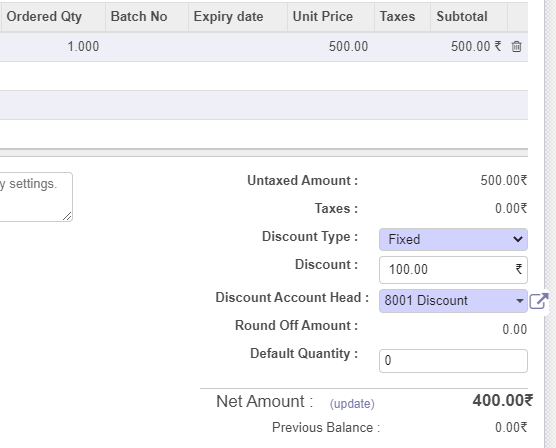# Odoo - Unable to remove discount after selecting no discount on Quotation.

After selecting a fixed discount, the discount amount will be deducted from the total amount. If we again select No discount and then Update, the total amount will not be equal to Subtotal, it is still the total amount with discount deducted.

First image with Fixed discount type.The second image with No Discount.Issue resolved by adding this code snippet in def onchange_discount in the /opt/bahmni-erp/bahmni-addons/bahmni_sale/models/sale_order.py#132-135

``````if self.discount_type == 'none':
self.discount_percentage = 0
self.discount = 0
``````

The onchange_discount function should be

``````@api.onchange('discount', 'discount_percentage', 'discount_type', 'chargeable_amount')
def onchange_discount(self):
amount_total = self.amount_untaxed + self.amount_tax
if self.chargeable_amount:
if self.discount_type == 'none' and self.chargeable_amount:
self.discount_type = 'fixed'
discount = amount_total - self.chargeable_amount
self.discount_percentage = (discount / amount_total) * 100
else:
if self.discount_type == 'none':
self.discount_percentage = 0
self.discount = 0
if self.discount:
self.discount_percentage = (self.discount / amount_total) * 100
if self.discount_percentage:
self.discount = amount_total * self.discount_percentage / 100``````
1 Like

@akshaybirajdar can you please raise a PR? @sushilp @ajeenckya can you please check the above?

@anandpatel

@akhilmalhotra @anandpatel can you review the PR please?

@akshaybirajdar Thanks for PR

@angshuonline PR looks fine, Please merge.

Thanks @sushantpatil1214 … btw, you can approve the same on github.Merged the PR